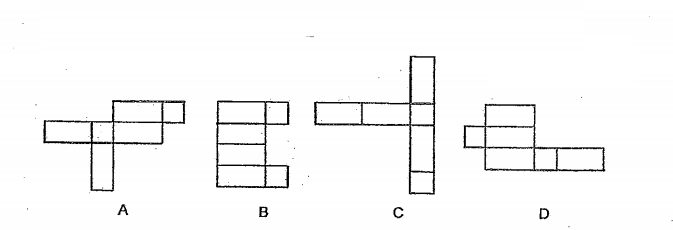# Primary 6 PSLE Practice #6

Time elapsed:
Q1. In the number line shown below, which value is closest to the reading at X?     [1 mark]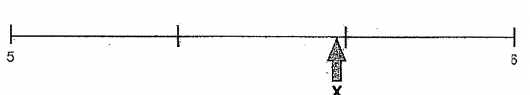Q2. At 11.00 a.m., a car left Town X for Town Y travelling at a speed of 80 km/h. At the same time, another car left Town Y for Town X travelling at a speed of 100 km/h. They did not change their speeds throughout. The distance between the two towns was 540 km. At what time did the cars pass each other?     [1 mark]
Q3. Elias had 24 more stamps than Natasha. When Natasha gave 18 stamps to Elias, Elias had 4 times as many stamps Natasha. How many stamps did Natasha have at first?     [2 marks]
Q4. What is the length of the pencil?     [1 mark]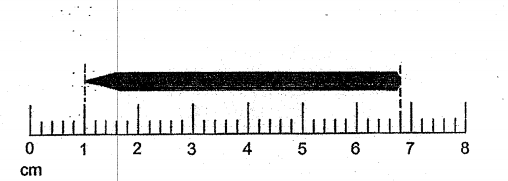Q5. In 1347.025, which digit is in the hundredths place?     [1 mark]
Q6. What is the value of 37 ÷ 21?     [1 mark]
Q7. A windmill turns 1500 rounds in a hour. How many rounds can it turn in 5 minutes?     [1 mark]
Q8. The average mass of Hannah, Vivian, and Hannah is 44 kg. Hannah is 6 kg heavier than Vivian and Vivian is 3 kg heavier than Hannah. What is Hannah' s mass?     [1 mark]
Q9. There were 60 more children in Room Y than in Room X. The number of boys in Room Y was 10 more than the number of boys in Room X. Given that there were 30 more girls than boys in Room X, how many more girls than boys were there in Room Y?     [2 marks]
Q10. Natasha cut out three identical right-angled triangles. She joined them to form the figure below. AB = 10 cm and AC = 6 cm. The perimeter of the figure is 56 cm. Find the area of Triangle ABC.     [2 marks]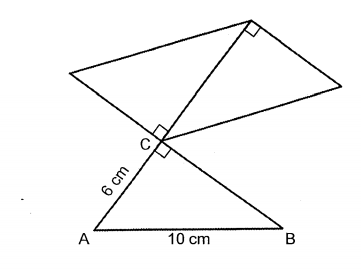Q11. In the diagram below, the shaded square is ___ of the mosque     [1 mark]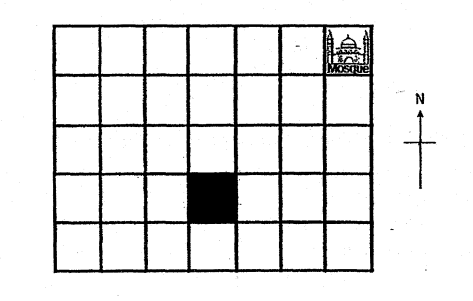Q12. Part of a scale is shown below. What is the value of the reading at X?     [1 mark]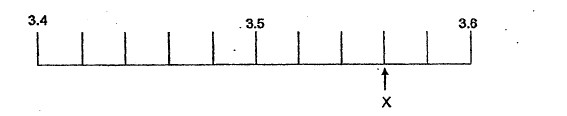Q13. How many eighths are there in 2 34?     [1 mark]
Q14. Francis took 2h 15min to bake a cake. He started baking at 11.35am. What time did he finish baking?     [1 mark]
Q15. There are twice as many boys as girls. There are twice as many adults as children. Which one of the following bar graph shows the above information correctly?     [1 mark]
Q16. Which of the following numbers is the largest?     [1 mark]
Q17. Zoe took a flight from 06 45 to 16 00. How long was the flight?     [1 mark]
Q18. In the figure, UTR and QTS are straight lines. SUQR is a trapezium. Which of the following statements is false?     [2 marks]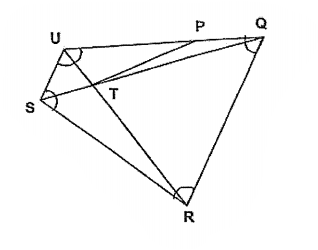Q19. Which one of the following is nearest to 1?     [1 mark]
Q20. Which of the following figures is net of a cuboid?     [1 mark]